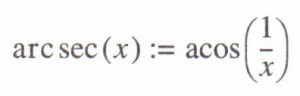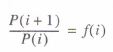# Using Functions and Variables MathCad Help

Mathcad’s symbolic processor does not treat functions and variables in exactly the same way as its numerical processor. These differences revolve around the answers to the following question:

• Does the symbolic processor “know” that a function or variable is defined elsewhere?

The answer to this depends on two things:

• Is the function or variable built-in or is it defined somewhere on the worksheet?
• Are you using the symbolic equal sign or a menu command?

The next two sections describe what Mathcad does with variables and functions in a symbolic transformation.

A related question is the converse. Symbolic transformations can sometimes return functions and constants which do not exist in Mathcad’s list of built-in functions and constants. These are described in the section “Special functions”.

Built-in Functions and Variables

As a general rule, built-in functions retain their meanings when used in symbolic transformations provided that it makes sense for them to do so. For example, functions like sin and log keep their meanings because these have a commonly accepted mathematical meaning. Other functions like linterp or rndlack any commonly accepted meaning so Mathcad doesn’t attempt to assign one.

Built-in functions that do retain their meanings when used in symbolic calculations include: trigonometric and hyperbolic functions and their inverses; logarithmic and exponential functions; the Re and 1m functions; the function; the T function; the mod function; <I>(the Heaviside step function); max and min; and the identity and eigenvals functions for matrices.

In general, these functions mean the same thing for both numerical evaluations and symbolic transformations. There are two subtle differences:

• Unlike the numerical mod function, the symbolic mod function requires an integer modulus, and can accept a polynomial as its first argument.
• Certain of the inverse trigonometric functions use different branches in the complex plane.

As a general rule, built-in constants also retain their meanings when used in symbolic transformations provided that it makes sense for them to do so. The symbolic processor will recognize π, e and 00 Moreover, these will have their exact meanings when used symbolically. When symbolic transformations are involved, there is no need to limit 00 to 10307 or to limit π to only fifteen digits of precision.

Built-in constants lacking an intuitive mathematical meaning are not recognized by the symbolic processor. For example, TOL and ORIGIN will not have their usual meanings in symbolic transformations. They will be treated like any other undefined variable. Figure 17-22 shows the difference in the way Mathcad treats functions in symbolic transformations. Note that the symbolic processor will recognize and evaluate the sin function, but when asked to evaluate rnd(3) the symbolic processor simply returns rnd(3) .

User-defined functions and variables

Functions and variables you define yourself are recognized by the symbolic processor when you use the symbolic equal sign discussed in the next section. They are not, however, recognized when you use Symbolics menu commands. Figure 17-22 shows the difference.

Special functions

A symbolic transformation sometimes comes back in terms of a function which isn’t part of Mathcad’s list of built-in functions. The list below gives definitions for those special functions. Except for Ei, erf, and Zeta, all of which involve infinite sums, and W, you can use these definitions to calculate numerical values. You can define many of these functions in Mathcad.

“Special Functions” topic in the Quicksheets of the Resource Center for examples.

y is Euler’s constant, approximately 0.5772156649.

The functions arcsec, arccsc, arccot, arcsech, arcscsh, arccoth can be calculated by taking reciprocals and using the Mathcad built-in functions acos, asin, etc. For example:The Psi function and T appear frequently in the results of indefinite sums and products. If you use a single variable name rather than a full range in the index placeholder of a summation or product, and you choose Evaluate Symbolically or one of the other symbolic evaluation commands, Mathcad will attempt to calculate an indefinite sum or product of the expression in the main placeholder. The indefinite sum of j(i) is an expression S(i) for which.

S(i + 1) -S(i) = I(i)

The indefinite product of j(i) is an expression P(i) for whichPosted on November 23, 2015 in Symbolic Calculation